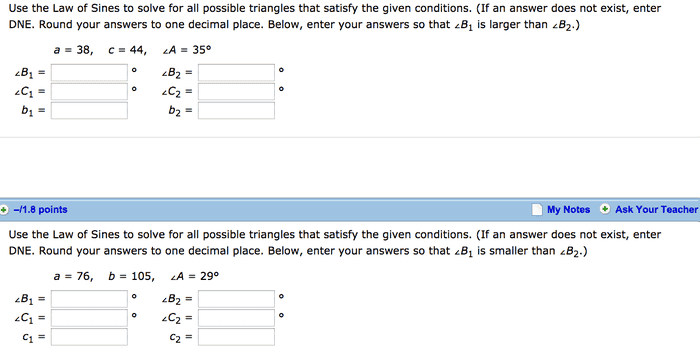# Help with law of sines/solving triangles

• Niaboc67

## Homework Statement## Homework Equations

SinA/a = SinB/b = SinC/c

## The Attempt at a Solution

a=38, c=44, ∠A = 35°
What I have so far:
sin(35)/38 = sinC/44
44sin(35) = 38sinC
44sin(35)/38 = sinC
sinC = 0.6641
sin^-1(0.6641)
∠C = 41.6º
Is this ∠C1?
After this I don't know how to derivive the rest.
---------------------------------------------
For the second problem:
a = 76, b = 105, ∠A = 29°
sin(29)/76 = sinB/105
105sin(29) = 76sinB
sinB = 105sin(29)/76
sin^-1(0.6698)
∠B = 42.1º
Again, after this point I am lost
I could really use some instruction on how to solve.
Thank you

If you know two angles of a triangle what do you know about the third angle?

Oh of course! Euclidean triangles equal 180º therefore for the first one 180-35-41.6 = 103.6º
and the second is 180-29-42.1 = 108.9
What must I figure out after this though?

Your choice of which angle is B is arbitrary right? So what would you choose as B1 so that B1 > B2 ?

If you mean where the angle is placed on the triangle, yeah I think it's arbitrary. If angle B1 is 103.6º and B2 must be larger than I suppose 105º? not sure if I am understanding the question here.

You only have one triangle colum one labels the angles one way and column two labels a different way.

Consider a 30-60-90 triangle and I say A is 90 then B is 30 or its 60 right and which ever you choose then C is the other angle. Your problem is constrained by the sin rule with A and a defined.